Calculus Volume 3

# Chapter 1

### Checkpoint

1.1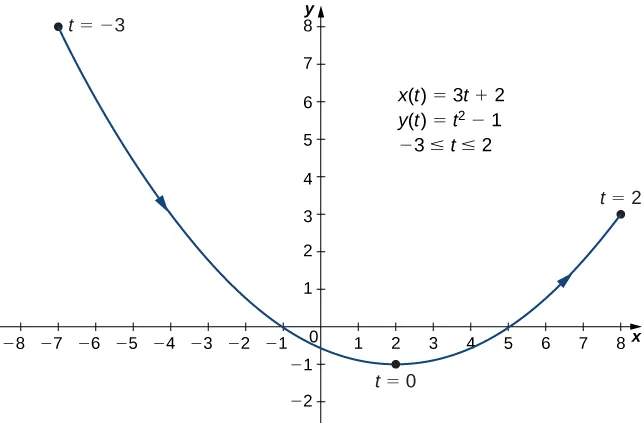1.2

$x=2+3y+1,x=2+3y+1,$ or $y=−1+3x−2.y=−1+3x−2.$ This equation describes a portion of a rectangular hyperbola centered at $(2,−1).(2,−1).$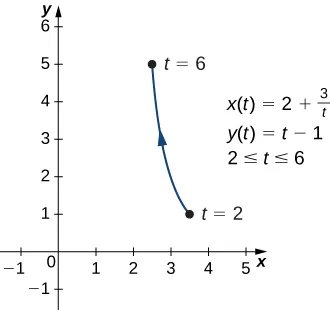1.3

One possibility is $x(t)=t,y(t)=t2+2t.x(t)=t,y(t)=t2+2t.$ Another possibility is $x(t)=2t−3,y(t)=(2t−3)2+2(2t−3)=4t2−8t+3.x(t)=2t−3,y(t)=(2t−3)2+2(2t−3)=4t2−8t+3.$

There are, in fact, an infinite number of possibilities.

1.4

$x′(t)=2t−4x′(t)=2t−4$ and $y′(t)=6t2−6,y′(t)=6t2−6,$ so $dydx=6t2−62t−4=3t2−3t−2.dydx=6t2−62t−4=3t2−3t−2.$
This expression is undefined when $t=2t=2$ and equal to zero when $t=±1.t=±1.$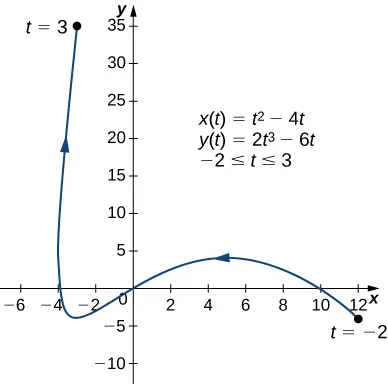1.5

The equation of the tangent line is $y=24x+100.y=24x+100.$

1.6

$d2ydx2=3t2−12t+32(t−2)3.d2ydx2=3t2−12t+32(t−2)3.$ Critical points $(5,4),(−3,−4),and(−4,4).(5,4),(−3,−4),and(−4,4).$

1.7

$A=3πA=3π$ (Note that the integral formula actually yields a negative answer. This is due to the fact that $x(t)x(t)$ is a decreasing function over the interval $[0,2π];[0,2π];$ that is, the curve is traced from right to left.)

1.8

$s = 2 ( 10 3 / 2 − 2 3 / 2 ) ≈ 57.589 s = 2 ( 10 3 / 2 − 2 3 / 2 ) ≈ 57.589$

1.9

$A = π ( 494 13 + 128 ) 1215 A = π ( 494 13 + 128 ) 1215$

1.10

$(82,5π4)(82,5π4)$ and $(−2,23)(−2,23)$

1.11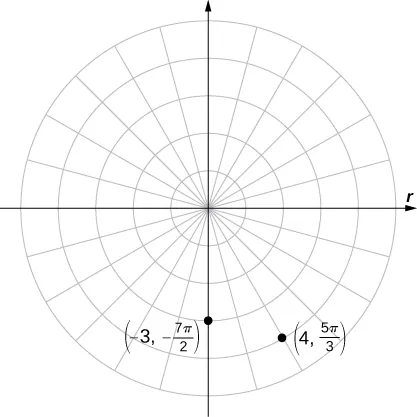1.12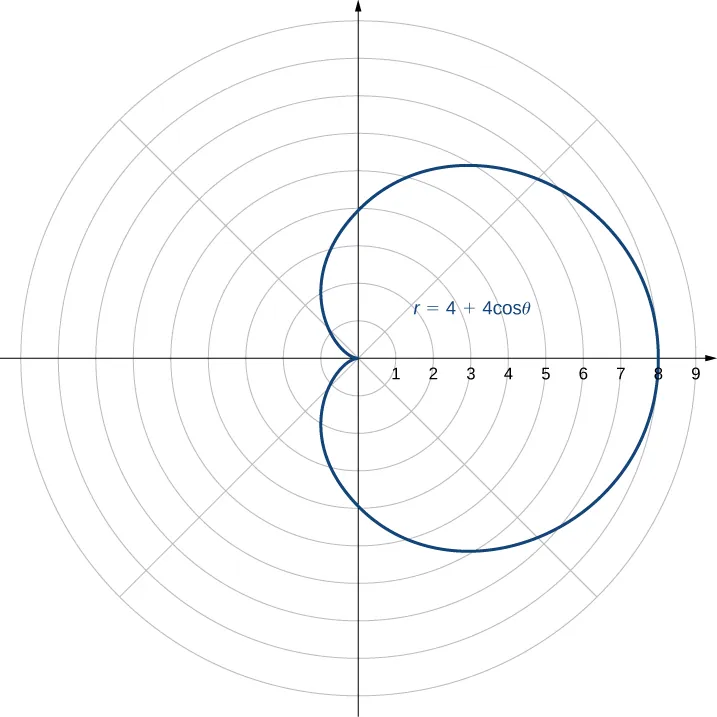The name of this shape is a cardioid, which we will study further later in this section.

1.13

$y=x2,y=x2,$ which is the equation of a parabola opening upward.

1.14

Symmetric with respect to the polar axis.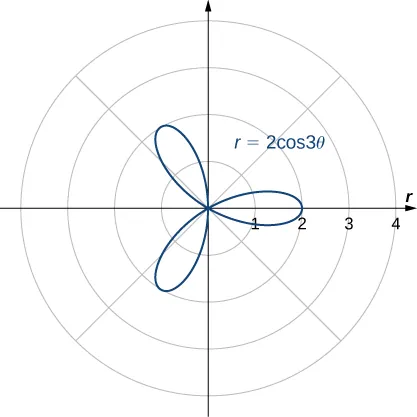1.15

$A = 3 π / 2 A = 3 π / 2$

1.16

$A = 4 π 3 + 2 3 A = 4 π 3 + 2 3$

1.17

$s = 3 π s = 3 π$

1.18

$x=2(y+3)2−2x=2(y+3)2−2$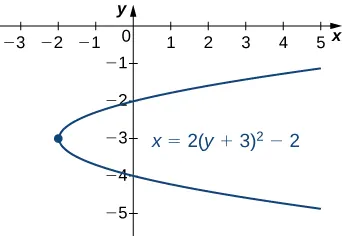1.19

$(x+1)216+(y−2)29=1(x+1)216+(y−2)29=1$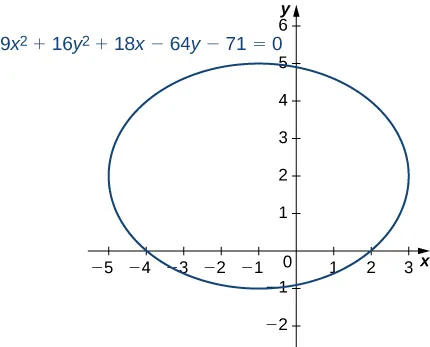1.20

$(y+2)29−(x−1)24=1.(y+2)29−(x−1)24=1.$ This is a vertical hyperbola. Asymptotes $y=−2±32(x−1).y=−2±32(x−1).$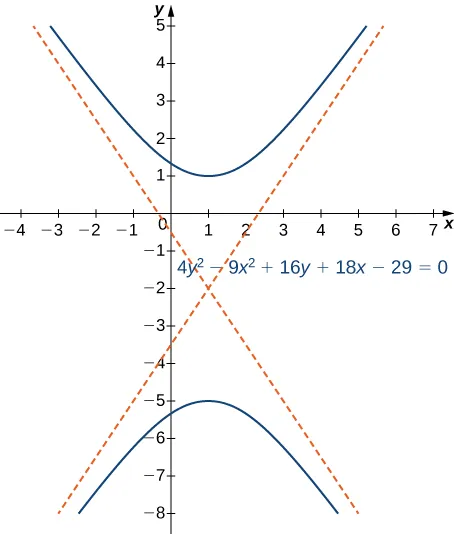1.21

$e = c a = 74 7 ≈ 1.229 e = c a = 74 7 ≈ 1.229$

1.22

Here $e=0.8e=0.8$ and $p=5.p=5.$ This conic section is an ellipse.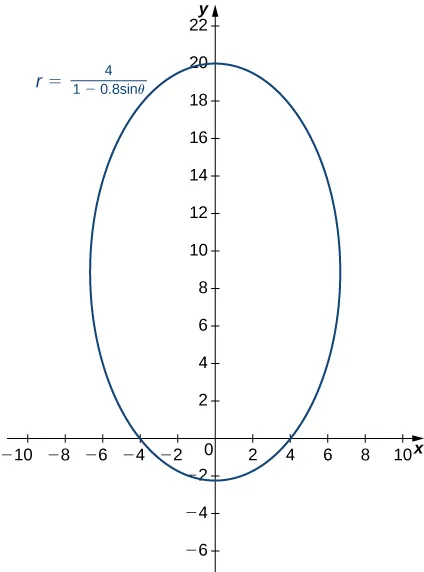1.23

The conic is a hyperbola and the angle of rotation of the axes is $θ=22.5°.θ=22.5°.$

### Section 1.1 Exercises

1.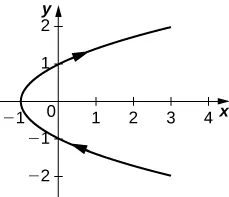orientation: bottom to top

3.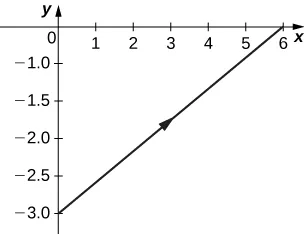orientation: left to right

5.

$y=x24+1y=x24+1$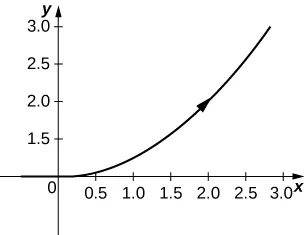7.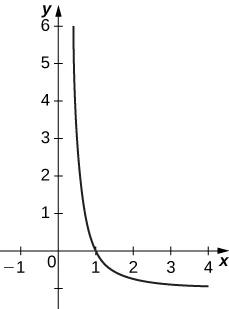9.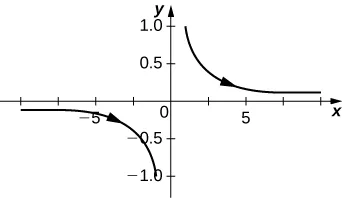11.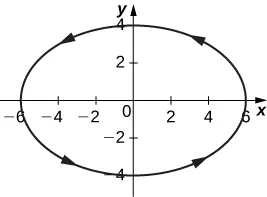13.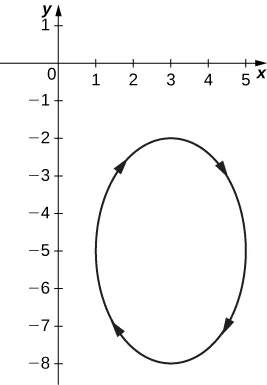15.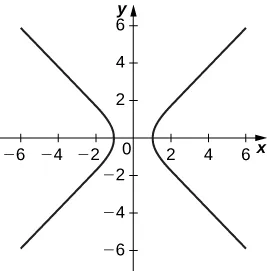Asymptotes are $y=xy=x$ and $y=−xy=−x$

17.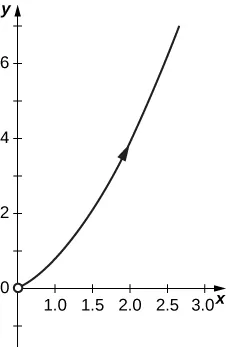19.21.

$y=x+12y=x+12$; domain: $x∈[–1,–∞).x∈[–1,–∞).$

23.

$x216+y29=1;x216+y29=1;$ domain $x∈[−4,4].x∈[−4,4].$

25.

$y=3x+2;y=3x+2;$ domain: all real numbers.

27.

$(x−1)2+(y−3)2=1;(x−1)2+(y−3)2=1;$ domain: $x∈[0,2].x∈[0,2].$

29.

$y=x2−1;y=x2−1;$ domain: $x∈(-∞,-1].x∈(-∞,-1].$

31.

$y2=1−x2;y2=1−x2;$ domain: $x∈[-1,1].x∈[-1,1].$

33.

$y=lnx;y=lnx;$ domain: $x∈[1,∞).x∈[1,∞).$

35.

$y=lnx;y=lnx;$ domain: $x∈(0,∞).x∈(0,∞).$

37.

$x2+y2=4;x2+y2=4;$ domain: $x∈[−2,2].x∈[−2,2].$

39.

line

41.

parabola

43.

circle

45.

ellipse

47.

hyperbola

51.

The equations represent a cycloid.53.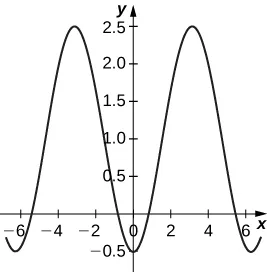55.

22,092 meters at approximately 51 seconds.

57.59.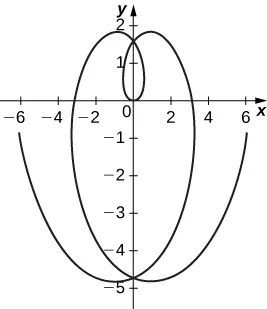61.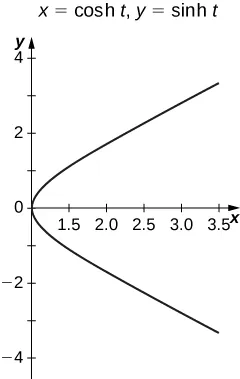### Section 1.2 Exercises

63.

0

65.

$−3 5 −3 5$

67.

$Slope=0;Slope=0;$ $y=8.y=8.$

69.

Slope is undefined; $x=2.x=2.$

71.

, $-45,85-45,85$.

73.

No points possible; undefined expression.

75.

$y = − ( 4 e ) x + 5 y = − ( 4 e ) x + 5$

77.

$y = –2 x + 3 y = –2 x + 3$

79.

$π 4 , 5 π 4 , 3 π 4 , 7 π 4 π 4 , 5 π 4 , 3 π 4 , 7 π 4$

81.

$d y d x = − tan ( t ) d y d x = − tan ( t )$

83.

$dydx=34dydx=34$ and $d2ydx2=0,d2ydx2=0,$ so the curve is neither concave up nor concave down at $t=3.t=3.$ Therefore the graph is linear and has a constant slope but no concavity.

85.

$dydx=4,d2ydx2=−63;dydx=4,d2ydx2=−63;$ the curve is concave down at $θ=π6.θ=π6.$

87.

No horizontal tangents. Vertical tangents at $(1,0),(−1,0).(1,0),(−1,0).$

89.

$− sec 3 ( π t ) − sec 3 ( π t )$

91.

Horizontal $(0,−9);(0,−9);$ vertical $(±2,−6).(±2,−6).$

93.

1

95.

0

97.

4

99.

Concave up on $t>0.t>0.$

101.

$e π 2 – 1 2 e π 2 – 1 2$

103.

$3 π 2 3 π 2$

105.

$6 π a 2 6 π a 2$

107.

$2 π a b 2 π a b$

109.

$1 3 ( 2 2 − 1 ) 1 3 ( 2 2 − 1 )$

111.

$7.075 7.075$

113.

$6 a 6 a$

115.

$6 2 6 2$

119.

$2 π ( 247 13 + 64 ) 1215 2 π ( 247 13 + 64 ) 1215$

121.

59.101

123.

$8 π 3 ( 17 17 − 1 ) 8 π 3 ( 17 17 − 1 )$

### Section 1.3 Exercises

125.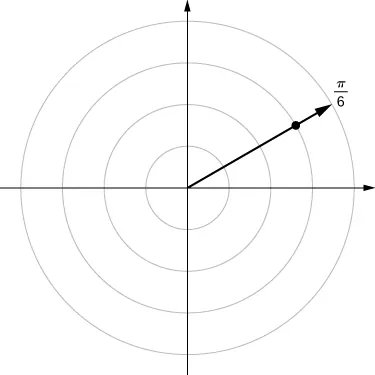127.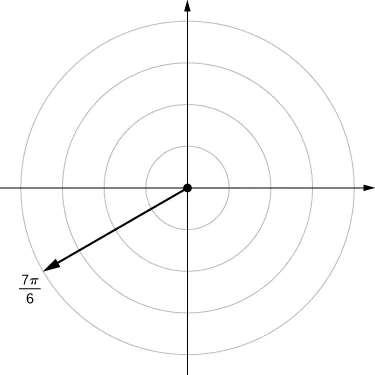129.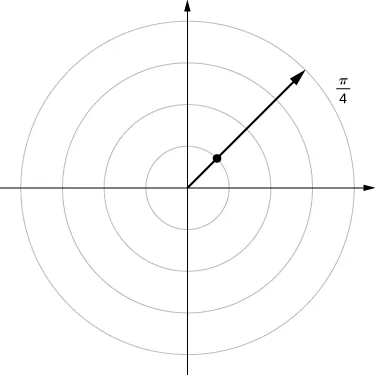131.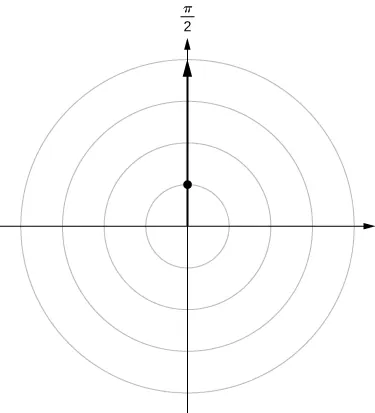133.

$B ( 3 , − π 3 ) B ( −3 , 2 π 3 ) B ( 3 , − π 3 ) B ( −3 , 2 π 3 )$

135.

$D ( 5 , 7 π 6 ) D ( −5 , π 6 ) D ( 5 , 7 π 6 ) D ( −5 , π 6 )$

137.

139.

141.

$( 2 3 , −5.759 ) ( −2 3 , 2.618 ) ( 2 3 , −5.759 ) ( −2 3 , 2.618 )$

143.

$( − 3 , −1 ) ( − 3 , −1 )$

145.

$( − 3 2 , −1 2 ) ( − 3 2 , −1 2 )$

147.

$( 0 , 0 ) ( 0 , 0 )$

149.

Symmetry with respect to the x-axis, y-axis, and origin.

151.

Symmetric with respect to x-axis only.

153.

Symmetry with respect to x-axis only.

155.

Line $y=xy=x$

157.

$y = 1 y = 1$

159.

Hyperbola; polar form $r2cos(2θ)=16r2cos(2θ)=16$ or $r2=16sec(2θ).r2=16sec(2θ).$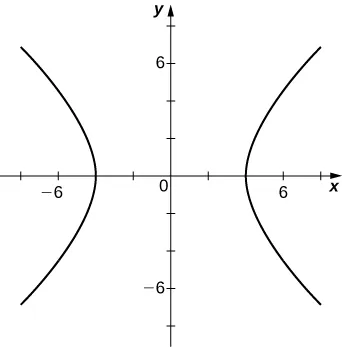161.

$r=23cosθ−sinθr=23cosθ−sinθ$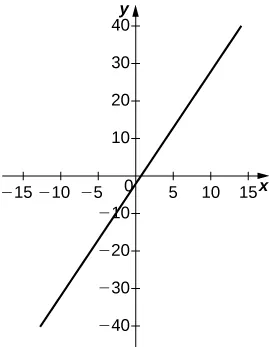163.

$x2+y2=4yx2+y2=4y$165.

$xtanx2+y2=yxtanx2+y2=y$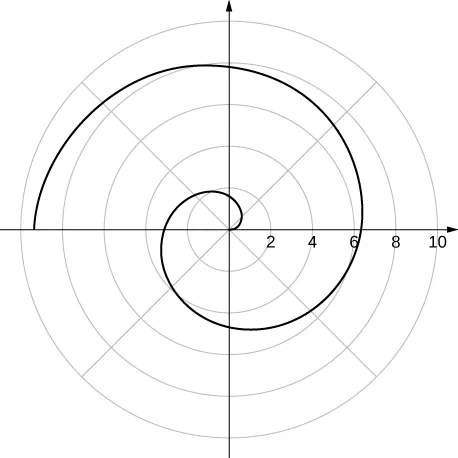167.y-axis symmetry

169.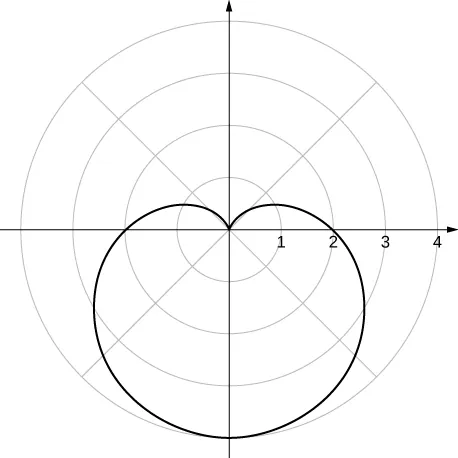y-axis symmetry

171.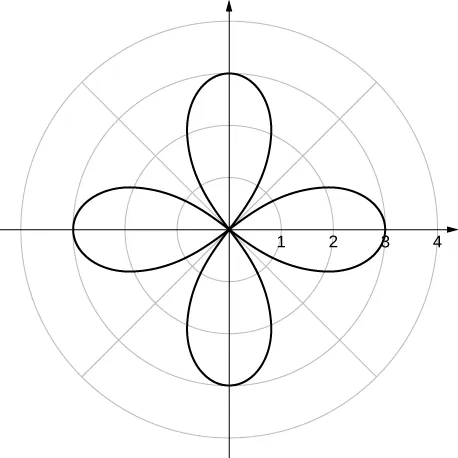x- and y-axis symmetry and symmetry about the pole

173.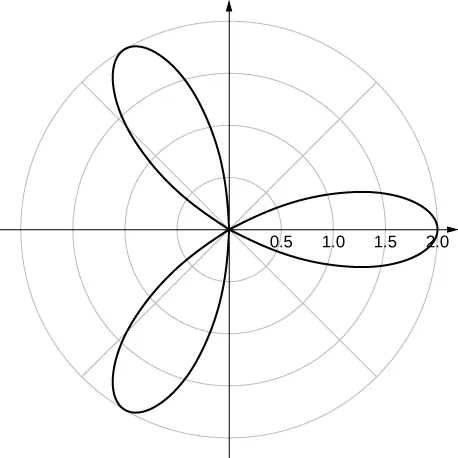x-axis symmetry

175.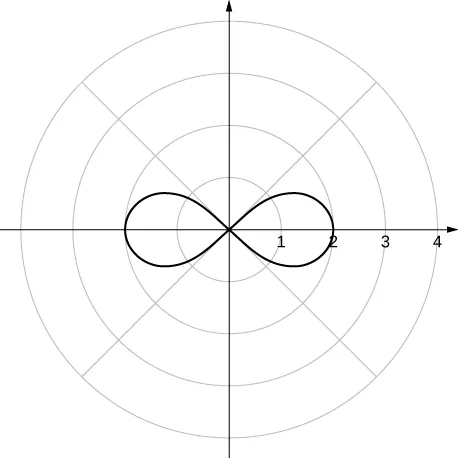x- and y-axis symmetry and symmetry about the pole

177.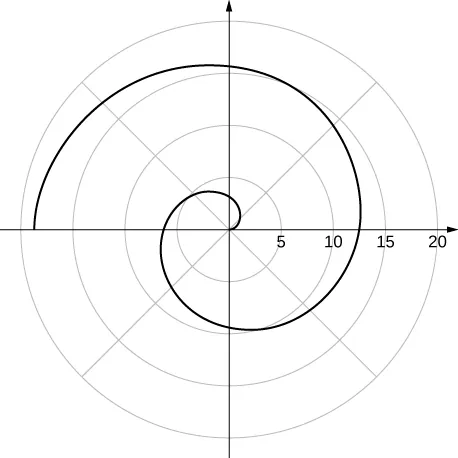no symmetry

179.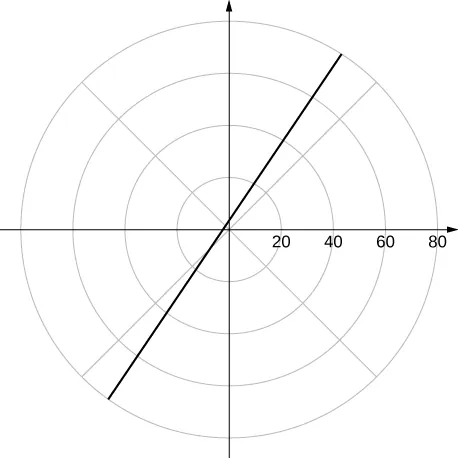a line

181.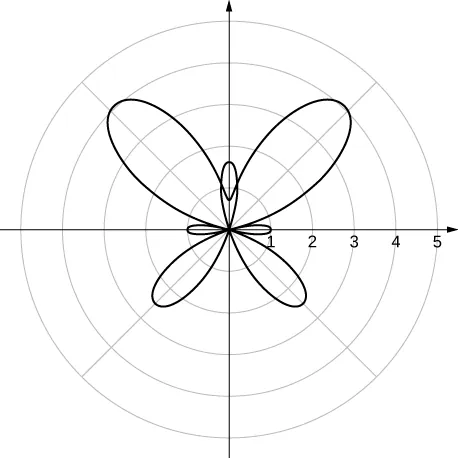183.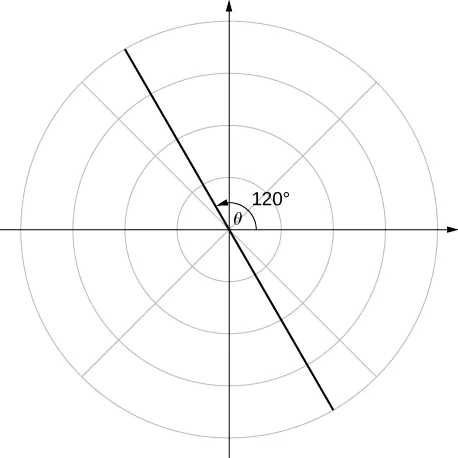185.187.

Answers vary. One possibility is the spiral lines become closer together and the total number of spirals increases.

### Section 1.4 Exercises

189.

$9 2 ∫ 0 π sin 2 θ d θ 9 2 ∫ 0 π sin 2 θ d θ$

191.

$32 ∫ 0 π / 2 sin 2 ( 2 θ ) d θ 32 ∫ 0 π / 2 sin 2 ( 2 θ ) d θ$

193.

$1 2 ∫ π 2 π ( 1 − sin θ ) 2 d θ 1 2 ∫ π 2 π ( 1 − sin θ ) 2 d θ$

195.

$∫ sin −1 ( 2 / 3 ) π / 2 ( 2 − 3 sin θ ) 2 d θ ∫ sin −1 ( 2 / 3 ) π / 2 ( 2 − 3 sin θ ) 2 d θ$

197.

$∫ π / 3 π ( 1 − 2 cos θ ) 2 d θ − ∫ 0 π / 3 ( 1 − 2 cos θ ) 2 d θ ∫ π / 3 π ( 1 − 2 cos θ ) 2 d θ − ∫ 0 π / 3 ( 1 − 2 cos θ ) 2 d θ$

199.

$4 ∫ 0 π / 3 d θ + 16 ∫ π / 3 π / 2 ( cos 2 θ ) d θ 4 ∫ 0 π / 3 d θ + 16 ∫ π / 3 π / 2 ( cos 2 θ ) d θ$

201.

$9 π 9 π$

203.

$9 π 4 9 π 4$

205.

$9 π 8 9 π 8$

207.

$18 π − 27 3 2 18 π − 27 3 2$

209.

$4 3 ( 4 π − 3 3 ) 4 3 ( 4 π − 3 3 )$

211.

$3 2 ( 4 π − 3 3 ) 3 2 ( 4 π − 3 3 )$

213.

$2 π − 4 2 π − 4$

215.

$∫ 0 2 π ( 1 + sin θ ) 2 + cos 2 θ d θ ∫ 0 2 π ( 1 + sin θ ) 2 + cos 2 θ d θ$

217.

$2 ∫ 0 1 e θ d θ 2 ∫ 0 1 e θ d θ$

219.

$10 3 ( e 6 − 1 ) 10 3 ( e 6 − 1 )$

221.

32

223.

6.238

225.

2

227.

4.39

229.

$A = π ( 2 2 ) 2 = π 2 and 1 2 ∫ 0 π ( 1 + 2 sin θ cos θ ) d θ = π 2 A = π ( 2 2 ) 2 = π 2 and 1 2 ∫ 0 π ( 1 + 2 sin θ cos θ ) d θ = π 2$

231.

$C = 2 π ( 3 2 ) = 3 π and ∫ 0 π 3 d θ = 3 π C = 2 π ( 3 2 ) = 3 π and ∫ 0 π 3 d θ = 3 π$

233.

$C = 2 π ( 5 ) = 10 π and ∫ 0 π 10 d θ = 10 π C = 2 π ( 5 ) = 10 π and ∫ 0 π 10 d θ = 10 π$

235.

$d y d x = f ′ ( θ ) sin θ + f ( θ ) cos θ f ′ ( θ ) cos θ − f ( θ ) sin θ d y d x = f ′ ( θ ) sin θ + f ( θ ) cos θ f ′ ( θ ) cos θ − f ( θ ) sin θ$

237.

The slope is $13.13.$

239.

The slope is 0.

241.

At $(4,0),(4,0),$ the slope is undefined. At $(−4,π2),(−4,π2),$ the slope is 0.

243.

The slope is undefined at $θ=π4.θ=π4.$

245.

Slope = −1.

247.

Slope is $−2π.−2π.$

249.

251.

Horizontal tangent at $(±2,π6),(±2,π6),$ $(±2,−π6).(±2,−π6).$

253.

Horizontal tangents at $π2,7π6,11π6.π2,7π6,11π6.$ Vertical tangents at $π6,5π6π6,5π6$ and also at the pole $(0,0).(0,0).$

### Section 1.5 Exercises

255.

$y 2 = 16 x y 2 = 16 x$

257.

$x 2 = 2 y x 2 = 2 y$

259.

$x 2 = −4 ( y − 3 ) x 2 = −4 ( y − 3 )$

261.

$( x + 3 ) 2 = 8 ( y − 3 ) ( x + 3 ) 2 = 8 ( y − 3 )$

263.

$x 2 16 + y 2 12 = 1 x 2 16 + y 2 12 = 1$

265.

$x 2 13 + y 2 4 = 1 x 2 13 + y 2 4 = 1$

267.

$( y − 1 ) 2 16 + ( x + 3 ) 2 12 = 1 ( y − 1 ) 2 16 + ( x + 3 ) 2 12 = 1$

269.

$x 2 16 + y 2 12 = 1 x 2 16 + y 2 12 = 1$

271.

$x 2 25 − y 2 11 = 1 x 2 25 − y 2 11 = 1$

273.

$x 2 7 − y 2 9 = 1 x 2 7 − y 2 9 = 1$

275.

$( y + 2 ) 2 4 − ( x + 2 ) 2 32 = 1 ( y + 2 ) 2 4 − ( x + 2 ) 2 32 = 1$

277.

$x 2 4 − y 2 32 = 1 x 2 4 − y 2 32 = 1$

279.

$e=1,e=1,$ parabola

281.

$e=12,e=12,$ ellipse

283.

$e=3,e=3,$ hyperbola

285.

$r = 4 5 + cos θ r = 4 5 + cos θ$

287.

$r = 4 1 + 2 sin θ r = 4 1 + 2 sin θ$

289.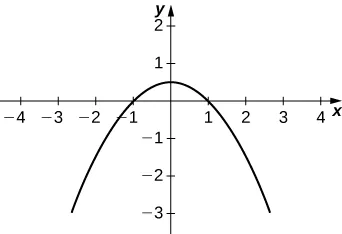291.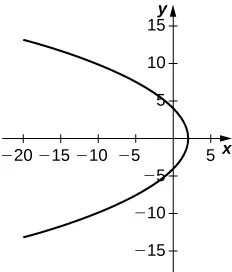293.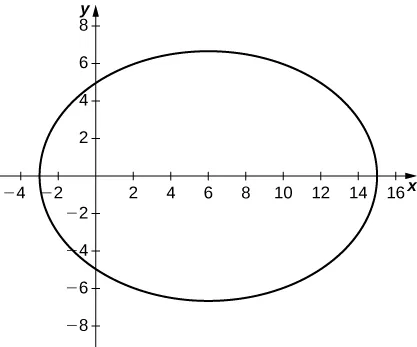295.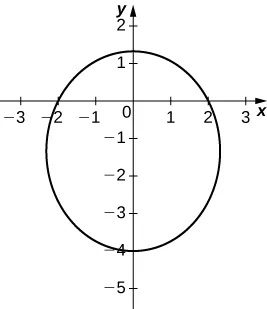297.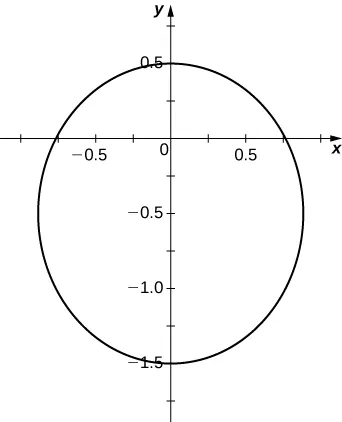299.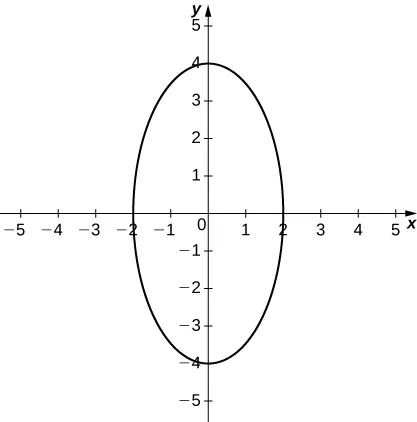301.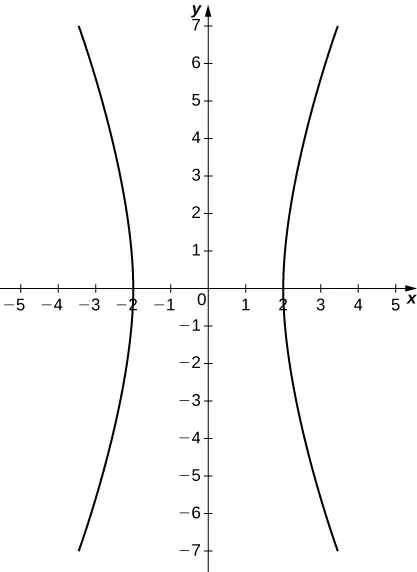303.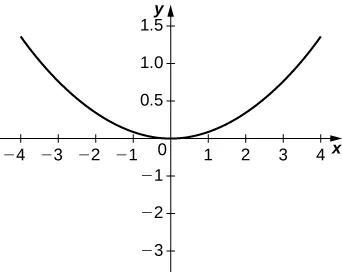305.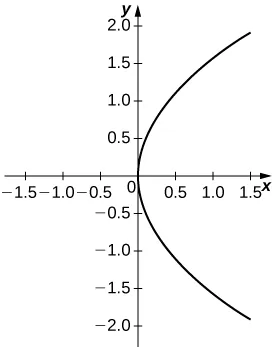307.

Hyperbola

309.

Ellipse

311.

Ellipse

313.

At the point 2.25 feet above the vertex.

315.

0.5625 feet

317.

Length is 96 feet and height is approximately 26.53 feet.

319.

$r = 2.616 1 + 0.995 cos θ r = 2.616 1 + 0.995 cos θ$

321.

$r = 5.192 1 + 0.0484 cos θ r = 5.192 1 + 0.0484 cos θ$

### Review Exercises

323.

True.

325.

False. Imagine $y=t+1,y=t+1,$ $x=−t+1.x=−t+1.$

327.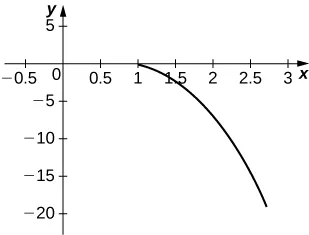$y=1−x3y=1−x3$

329.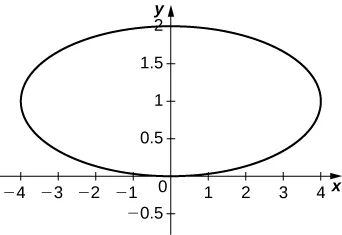$x216+(y−1)2=1x216+(y−1)2=1$

331.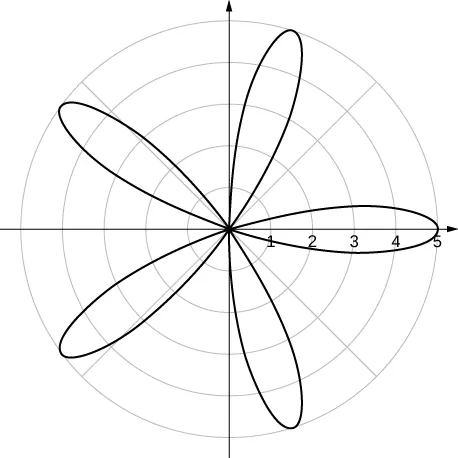333.

$r 2 = 4 sin 2 θ − cos 2 θ r 2 = 4 sin 2 θ − cos 2 θ$

335.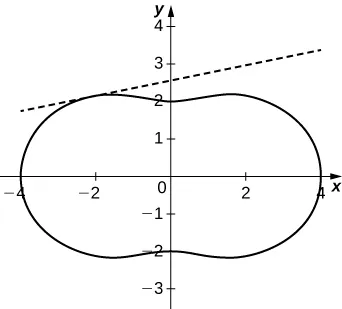$y=322+15(x+322)y=322+15(x+322)$

337.

$e 2 2 e 2 2$

339.

$9 10 9 10$

341.

$( y + 5 ) 2 = −8 x + 32 ( y + 5 ) 2 = −8 x + 32$

343.

$( y + 1 ) 2 16 − ( x + 2 ) 2 9 = 1 ( y + 1 ) 2 16 − ( x + 2 ) 2 9 = 1$

345.

$e=23,e=23,$ ellipse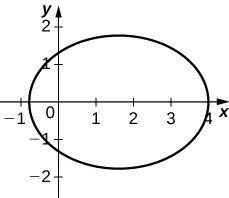347.

$y219.032+x219.632=1,y219.032+x219.632=1,$ $e=0.2447e=0.2447$

Order a print copy

As an Amazon Associate we earn from qualifying purchases.